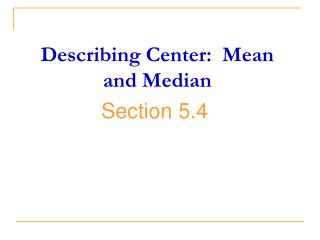# Describing Center: Mean and Median - PowerPoint PPT PresentationDownload PresentationDescribing Center: Mean and Median

Describing Center: Mean and MedianDownload Presentation## Describing Center: Mean and Median

- - - - - - - - - - - - - - - - - - - - - - - - - - - E N D - - - - - - - - - - - - - - - - - - - - - - - - - - -
##### Presentation Transcript

1. Describing Center: Mean and Median Section 5.4

2. Chapter 5: Exploring Data: DistributionsDescribing Center: Mean and Median Look at table 5.2 at the right. We are going to make the dotplot of the city gas mileages of midsize cars.

3. Chapter 5: Exploring Data: DistributionsDescribing Center: Mean and Median Two Most Common Ways to Describe the Center: Mean and Median 1. Mean “average value” Ordinary arithmetic average of a set of observations, average value. To find mean of a set of observations, add their values, (x1, x2, x3, …, xn) and divide by the number of observations, n. = (x1 + x2 + … +xn)/n

4. Chapter 5: Exploring Data: DistributionsDescribing Center: Mean and Median Example 1 Find the mean city mileage for the 13 midsize cars in Table 5.2

5. Chapter 5: Exploring Data: DistributionsDescribing Center: Mean and Median Suppose we take the outlier (Toyota Prius) out of the data. What would the mean city mileage be?

6. Chapter 5: Exploring Data: DistributionsDescribing Center: Mean and Median The single outlier adds more than 2 mpg to the mean city mileage. This illustrates an important weakness of the mean as a measure of center: The mean is sensitive to the influence of a few extreme observations. These may be outliers, but a skewed distribution that has no outliers will also pull the mean toward its long tail.

7. Chapter 5: Exploring Data: DistributionsDescribing Center: Mean and Median 2. Median “middle value” The midpoint or center of an ordered list; middle value of a set of observations; half fall below the median and half fall above. Arrange observations in increasing order (smallest to largest). If the number of observations is odd, the median M is the center observation in the ordered list. If the number of observations is even, the median M is the average of the two center observations in the ordered list. The position of the median is (n + 1)/2.

8. Chapter 5: Exploring Data: DistributionsDescribing Center: Mean and Median Example 2 Find the median of city mileage of the 13 midsize cars. 1. Arrange the data in increasing order. 15 16 18 19 20 20 21 21 21 22 24 27 48 2. Since the number of observations is odd, the median position is (13 + 1)/2 = 7th observation. 21

9. Chapter 5: Exploring Data: DistributionsDescribing Center: Mean and Median What is the median if we drop the Toyota Prius? 15 16 18 19 20 20 21 21 21 22 24 27 Since the number of observations is even, the median position is (12 + 1)/2 = 6.5 which means it is the mean of the 6th and 7th observations. (20 +21)/2 = 20.5

10. Chapter 5: Exploring Data: DistributionsDescribing Center: Mean and Median You see that the median resists the influence of extreme observations better than the mean does. A very high value like the Toyota Prius is simply one observation to the right of center and removing it hardly changed the median at all. In fact, removing an extreme outlier can leave the median completely unchanged while significantly changing the mean

11. Chapter 5: Exploring Data: DistributionsDescribing Center: Mean and Median Another common numerical summary of a distribution is the 3. Mode – the most frequently occurring value. Like the mean and median, it is a measure of location for a distribution. However, the mode is not necessarily a good measure of center.

12. Chapter 5: Exploring Data: DistributionsDescribing Center: Mean and Median Example 3 A. Find the mode of the city mileage in table 5.2. The mode is 21 since it occurs 3 times. B. Find the mode of the highway mileage in table 5.2. The modes are 27, 29, 30, 33.

13. Chapter 5: Exploring Data: DistributionsDescribing Center: Mean and Median We can also identify the mode(s) of a histogram. The mode of this histogram is the class \$34,000 to \$38,000 since it is the tallest.